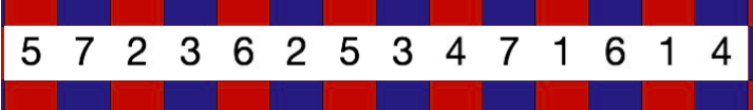#### You may also like### Calendar Capers

Choose any three by three square of dates on a calendar page...Make a set of numbers that use all the digits from 1 to 9, once and once only. Add them up. The result is divisible by 9. Add each of the digits in the new number. What is their sum? Now try some other possibilities for yourself!### Doodles

Draw a 'doodle' - a closed intersecting curve drawn without taking pencil from paper. What can you prove about the intersections?

# More Number Sandwiches

##### Age 11 to 16 Challenge Level:

Thank you to Ashlynn from ISF in Hong Kong, who sent in a full solution. This is Ashlynn's work:In a "7-­sandwich", how many red squares are covered and how many blue squares are
covered?

There are 7 red squares covered and 7 blue squares covered.

If it were possible to make a "6­-sandwich", how many red squares and how many blue
squares would be covered?

For a 6­-sandwich, total there are 12 squares covered. 6 are red, 6 are blue.

If you place a 1 on a blue square, on which colour will you place the other 1?
Blue

If you place a 2 on a blue square, on which colour will you place the other 2?
Red

If you place a 3 on a blue square, on which colour will you place the other 3?...
Blue

In general, what can you say about the colours on which you place pairs of numbers?
If it is an odd number, it covers the same colour of tile. e.g. Red­-Red, Blue-­Blue
If it is an even number, it covers different colours of tile. e.g. Red­-Blue, Blue-­Red

When you try to make a sandwich with the numbers from 1 to 5, or from 1 to 6, what goes wrong?
For a 6­-sandwich, in total there should be 12 tiles covered. 6 are red tiles, 6 are blue tiles.
We try:
Number Possible Colours of Tiles Covered
1 R-R
2 R-B
3 R-R
4 R-B
5 B-B
6 IMPOSSIBLE!

It is impossible because in 6­-sandwich there are 3 even numbers which cover 3R and 3B.
Odd numbers can only cover an even number of R or B. They cannot make another 3R or 3B.

For a 5-­sandwich, in total there should be 10 tiles covered. 5 are red tiles, 5 are blue tiles.
We try:
Number Possible Colours of Tiles Covered
1 R-R
2 R-B
3 B-B
4 R-B
5 IMPOSSIBLE!

It is impossible because in 5­-sandwich there are 2 even numbers which cover 2R and 2B.
Odd numbers can only cover an even number of R or B. They cannot make another 3R or 3B.

Which other sandwiches are impossible? How can you be sure?
Odd numbers can only cover an even number of R or B.
 Number Sandwich Number of    Red and  Blue Need to be Covered Even numbers in the sandwich No. of R and B covered by even number No. of R and B needed to be covered by odd numbers POSSIBLE? 1 NO 2 2R, 2B 2 1R, 1B 1R, 1B NO 3 3R, 3B 2 1R, 1B 2R, 2B YES 4 4R, 4B 2, 4 2R, 2B 2R, 2B YES 5 5R, 5B 2, 4 2R, 2B 3R, 3B NO 6 6R, 6B 2, 4, 6 3R, 3B 3R, 3B NO 7 7R, 7B 2, 4, 6 3R, 3B 4R, 4B YES 8 8R, 8B 2, 4, 6, 8 4R, 4B 4R, 4B YES 9 9R, 9B 2, 4, 6, 8 4R, 4B 5R, 5B NO 10 10R, 10B 2, 4, 6, 8, 10 5R, 5B 5R, 5B NO 11 11R, 11B 2, 4, 6, 8, 10 5R, 5B 6R, 6B YES 12 12R, 12B 2, 4, 6, 8, 10, 12 6R, 6B 6R, 6B YES 13 13R, 13B 2, 4, 6, 8, 10, 12 6R, 6B 7R, 7B NO 14 14R, 14B 2, 4, 6, 8, 10, 12, 14 7R, 7B 7R, 7B NO 15 15R, 15B 2, 4, 6, 8, 10, 12, 14 7R, 7B 8R, 8B YES

We can see a pattern:
If the number sandwich is an even number. When divided by 2, if the quotient is odd number, then it is impossible to make the number sandwich.

If the number sandwich is an odd number. When [decreased] by 1 and then divided by 2, if the quotient is odd number, then it is impossible to make the number sandwich.